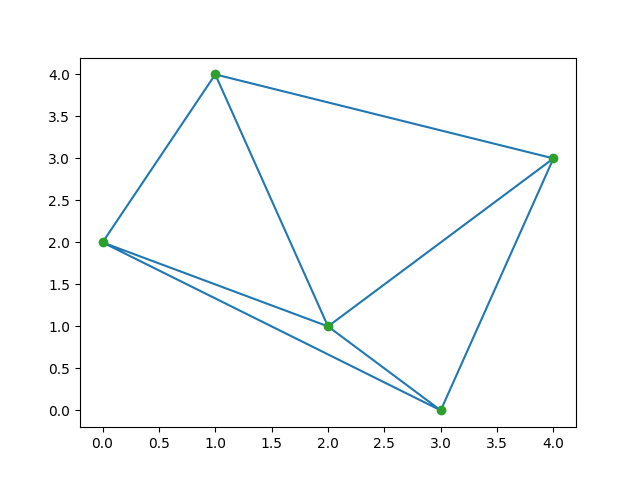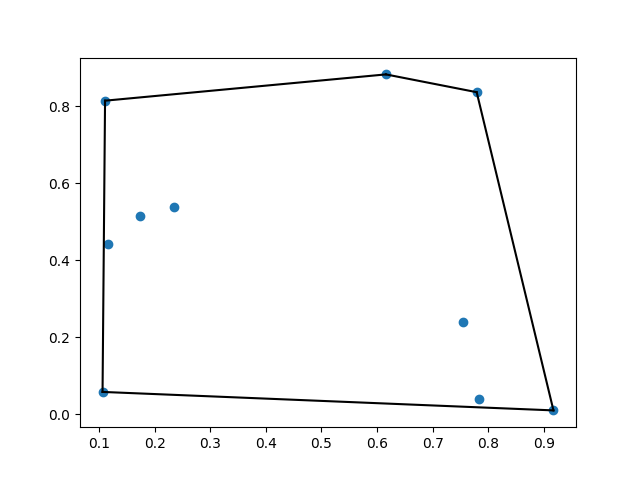scipy.spatial – Spatial data structures and algorithms

• Difficulty Level : Medium
• Last Updated : 17 Oct, 2021

In this article, we are going to see spatial data structure and algorithms, it is used to represent data in a geometric space.

What is spatial data structure?

The spatial package computes the triangulations, Voronoi diagrams, and convex hulls of a set of points, by leveraging the Qhull library. Moreover, it contains KDTree implementations for nearest neighbor point queries and utilities for distance computations in various metrics.

Example 1: Delaunay Triangulations

In mathematics and computational geometry, a Delaunay triangulation for a given set p of discrete points, in a plane is a triangulation DT(p) such that no point p is inside the circumcircle of any triangle in DT(p).

Python

 from scipy.spatial import Delaunayimport numpy as npimport matplotlib.pyplot as plt  points = np.array([[1, 4], [2, 1], [3, 0],                    [0, 2], [4, 3]])tri = Delaunay(points)  plt.triplot(points[:, 0], points[:, 1], tri.simplices.copy())plt.plot(points[:, 0], points[:, 1], 'o')plt.show()

Output:Example 2: Coplanar points

Coplanar points are three or more points that lie in the same plane. Recall that, a plane is a flat surface, which extends without end in all directions.

Python

 from scipy.spatial import Delaunayimport numpy as np  points = np.array([[0, 0], [0, 1], [1, 0],                    [1, 1], [1, 1]])tri = Delaunay(points)print(tri.simplices)print(tri.coplanar)

Output:

[[3 1 0]
[2 3 0]]
[[4 0 3]]

Example 3: Convex Hulls

The convex hull or convex envelope of a set of points X in the euclidean space(or, more generally in affine space over the reals) is the smallest convex set that contains X.

Python

 from scipy.spatial import ConvexHullimport numpy as npimport matplotlib.pyplot as plt  points = np.random.rand(10, 2)hull = ConvexHull(points)  plt.plot(points[:, 0], points[:, 1], 'o')for simplex in hull.simplices:    plt.plot(points[simplex, 0], points[simplex, 1], 'k-')  plt.show()

Output:Example 4: KPTrees

kd-tree is a quick nearest-neighbor lookup. And Kdtree() methods return the kd-tree object

Python3

 from scipy.spatial import KDTree  points = np.random.rand(10, 2)kdtree = KDTree(points)result = kdtree.query((1, 1))print(result)

Output:

(0.5144859720297681, 9)

Attention geek! Strengthen your foundations with the Python Programming Foundation Course and learn the basics.

To begin with, your interview preparations Enhance your Data Structures concepts with the Python DS Course. And to begin with your Machine Learning Journey, join the Machine Learning - Basic Level Course

My Personal Notes arrow_drop_up